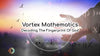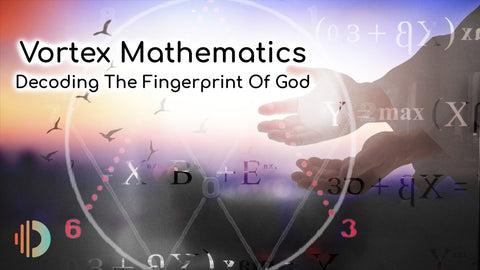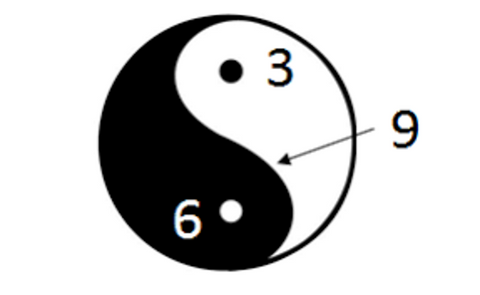• check_circle Body Tested
• check_circle Everlasting
• check_circle Easy To Apply

Balanced Life Energy | 30-Day free returns

Get in touch with us

# Vortex Mathematics: Decoding The Fingerprint Of God“Number rules the universe. Number is the ruler of forms and ideas, and the cause of gods and demons.”
- PythagorasAs humans, our pursuit to understand the true nature of reality has led us to unbelievable discoveries across science and spirituality. Each new revelation takes us one step closer to the fundamental truth - and a basic understanding of vortex math is crucial to this journey.

If you’ve read our numerology blog and sacred geometry beginners guide, then you’ll see how those concepts naturally express themselves in vortex mathematics. Vortex math gives us the numerical tools we need to dig deeper into the nature of creation and consciousness.

Today, we will take you through the building blocks of vortex math, and what it tells us about the nature of both our consciousness and the cosmos.

## What Is Vortex Math?

Vortex-based mathematics is a dynamic form of math that shows us the relationship between and qualities of numbers rather than only the quantity they represent. Found and popularized by Marko Rodin, vortex math is a divine algorithm that explains the underlying blueprint of the universe.

It also numrically expresses the relationship between the material, the ether and the zero point. These are important themes in Omnia's science which help us to understand why wireless radiation is discordant with the vibration of the human body.

We’ve said this before, and we’ll say it again: numbers and math were not ‘invented’ by humans - they were merely discovered. Numbers are alive in themselves, and have their own intrinsic, universal meanings.

Mathematics is the language of creation - it forms the basis of everything we can (and cannot) see. Everything in nature expresses itself via numbers, and we have only assigned certain symbols that represent these fundamental quantities.

According to the founder of vortex math, this system reveals the pathways that all energy takes to assume various forms - right from the quantum level (inner structure of an atom) to cosmic proportions (galaxies, black holes). Understanding vortex math is key to uniting the micro and the macro scales of our reality.

## Vortex Math & The Torus

As we go deeper into the calculations of vortex-based mathematics and the visual patterns they generate, you will see that it gradually forms a three-dimensional numerical matrix. And this 3D grid is shaped in the form of a torus - a donut-like shape achieved when a circle is rotated in a 3D space about a straight line that does not pass through the circle itself.

But what is so special about the torus shape?  A lot! As Omnia's science explains, the atom is a torus field of light which follows two phases: the light ring compresses itself into matter and then discharges itself into the ether, into empty space.

We will explain below how this is mirrored in vortex math.

The natural universe is therefore built on the torus shape. This was always the truth, since way before we discovered it. It is a self-sufficient, coherent, unified shape and its past and future blend into each other through the interchange between the cycles of compression (making) and expansion (discharging) that the torus field of light in the atom obeys. This geometrical form repeats both the beginning and the end in a spinning, vibrational cycle.

The earth’s magnetic field is shaped like a torus, and so is the energy  field around each human being which emanates from the human heart. In fact, human DNA is derived from a toroid shape, and physicists recently discovered a torus structure surrounding a supermassive black hole.

But that’s not all. Vortex-based math tells us that the torus is the shape of both the atom and our entire universe. It is the essence found in both the micro and the macro scales.

## Digital Root & Doubling

From childhood, we are taught the base 10 number system or the decimal system, which was originally founded in ancient India. The base 10 system uses the numbers 0 to 9 to assign certain place values to every number. But vortex math delves deeper into the hidden organisation and relationship behind each of these numerical symbols.

The key to understanding vortex math starts with the concept of ‘digital root’ and doubling. Read our numerology blog for a detailed explanation of the digital root). A digital root is found by adding all the digits in a number until we are left with only a single digit.

For eg. let’s take the digital root of the number 381

381 = 3 + 8 + 1 = 12

12 = 1 + 2 = 3

The digital root of 381 is 3.

Vortex-based math reveals the underlying intelligence that guides all order in the universe. We can also call it the ‘mathematical fingerprint of god’, since this pattern (both numerical and visual) can be seen all around us, in every corner of the cosmos.

To find this numerical fingerprint, we begin with the principle of doubling, starting with 1:

1, 2, 4, 8, 16, 32, 64, 128 and so on.

### Why only doubling?

All matter is energy (or frequency, vibration, light), and one octave represents the overall energy in a specific frequency range. Octaves show us the relationship between frequencies, and the growth of octaves happens by doubling of frequency.

The enigmatic genius Walter Russell’s version of the periodic table showed that there are 9 different ‘octaves’ or ‘pressure states’ found in nature. Each element in the periodic table falls within these 9 octaves. Just like an endless river, each element in the periodic table ‘flows’ from its preceding element. Russell showed that “all 9 octaves constitute one complete transfer cycle of the universal constant of energy into all dimensions in space.”

Note that the number '9' is an all-powerful number in this system.

In simpler words - all matter is light - and everything is made of sound, frequency, and vibration!## The Fundamentals Of Vortex Math

Now, let’s explain the crucial pillars of vortex mathematics:

### The 1,2,4,8,7,5,1, Sequence

To do this, let us start with a circle, and divide its circumference into 9 equal parts - and numbering each point from 1 to 9 in the following fashion:Now, let us begin doubling the numbers:

Doubling that, we get 2

Next, we get 4

Doubling 4, we get 8

8+8= 16 = 1+6 = 7

16+ 16 = 32 = 3+2 = 5

32 + 32 = 64 = 6+4 = 10, 1+ 0 = 1

If we carry on this process, we will see that the sequence keeps endlessly repeating:

1, 2, 4, 8, 7, 5 and back to 1.

Now, let’s go back to our circle - and you’ll see one side bearing the numbers 1, 2 and 4, while the other half bears 8, 7, and 5. if you trace these numbers in this order within our circle, you will get a 'figure of 8', which is the infinity sign. And thus, this sequence represents creation itself.

## 3, 6 and 9: the Ether

But what about 3, 6 and 9 - the numbers that are missing from this sequence?Look deeper and you’ll find that the 1,2,4,8,7,5,1 sequence is governed by the numbers 3 and 6! In vortex mathematics, 3 and 6 represent the yin/yang balance, flowing in opposite directions to maintain the balance.

Take the sum of any two numbers directly connected to each other in our circle:

5 + 1 = 6

2 + 1 = 3

4 + 2 = 6

7+5 = 12, 1 + 2 = 3

8+7 = 15, 1 + 5 = 6

8+4 = 12, 1+ 2 = 3

We get the alternate sequence 3,6,3,6,3,6….until infinity!

This alternate 3,6,9 sequence represents the discharge of energy into ether - or the invisible energy field permeating through the entire cosmos.

So just as the number sequence 1,2, 4, 8, 7, 5, 1.. represents the material world because it represents the spin of energy in the torus field of each atom, we have its counterpart, the ether, expressed as the 3, 6 and the 9.

And that leaves one digit which is: zero. Note how the zero is in the middle of the diagram. The zero point is effectively the point from which all matter expresses itself and it sits at the centre of the spinning ring of light (the 'figure of 8').

## The Number 9 - The Fingerprint Of God

After understanding the above two sequences, we are left with a single number missing from both - the number 9.

In vortex-based mathematics, 9 is the most important number. It is considered the mathematical fingerprint of God, and the number of the spirit.

In the circle diagram that we started with, the number 9 is at the top - balancing both sides of the circle. This is why it is called the ‘node’ - an omnipotent symbol that represents consciousness and enlightenment.

In Hinduism, the number 9 is considered to be complete, perfect and divine, since it represents the end of the decimal system. 9 has also been revered across several ancient cultures - from Norse mythology to ancient Egypt to Chinese culture.

So while 3 and 6 represent the yin/yang duality, the number 9 is the ‘S’ curved shape that both connects and separates both sides.## Some more 'Coincidences'.

If you add up all the numbers in the sequence (9+1+2+3+4+5+6+7+8), this comes to 45 and this has a digital root of 9

If you add the infinity flow numbers (1+2+4+8+7+5), this comes to 27 and this has a digital root of 9

If you take each row of numbers on opposite sides of the circle, you also get the number 9

8+1

7+2

5+4

So the 9 can be said to be the master, 'self-organising' number. Could it be that the number 9 also denotes balance

## Vortex Math & Omnia’s Science

The conclusions drawn from vortex math resonate with the system devised by Dr. Ilija Lakicevic, upon which Omnia’s science has been founded. According to this system, we find that:

• The atom is a torus-shaped field of light that spins itself into 'matter'. The spin of energy follows a figure of 8, or 'infinity'-shaped flow as the torus is materialised.

• At the centre of the torus field and therefore at the centre of each atom sits a magnetic, still point. This is the 'zero point' (the number zero in all the diagrams above). The zero point is magnetic and holds the electric current of light in its torus shape and in balance.
• The 1,2,4,8,7,5,1 sequence represents compression of the light ring into matter, depicting the creation of our physical reality: the 'visible', material world.
• The 3,6,9 represents the ether, into which the light ring is discharged. It depicts expansion, our metaphysical reality and the 'invisible'.

And together, these numerical sequences and the mathematical relationships that govern them bring forth matter from a higher dimensional realm into our physical three-dimensional reality. We can see how elegantly vortex math explains the true nature of the atom and the cyclical nature in which its energy compresses and expands.

## Final Thoughts

So, what do the secrets revealed by vortex math mean for us? The realizations can be different for each of us, but the biggest takeaway is this - all matter around and beyond us are different forms of the same energy. From the black holes to the microscopic atom - the fundamental structure of all creation is one and the same.

By gazing into the blueprint of divine creation, we can decode how this energy behaves. We can do it through geometry, through music, thround Physics (the right concepts in physics) and we can do it through vortex math.

In vortex math we see how the beginning and the end, or the 'material' and the 'etheric' cycle, are inextricably intertwined with each other. This means that there is so much more that unites us all, and that any division or separation is merely an illusion. In fact, it is a false reality.

What will you do with this sacred realization? This is a planet of free will and therefore the choice is yours.

https://qz.com/837742/shapes-in-design-the-torus-is-the-design-worlds-next-trendy-shape/

https://en.wikipedia.org/wiki/Tesla_coil

https://en.wikipedia.org/wiki/9

https://scitechdaily.com/astronomers-observe-doughnut-shaped-torus-surrounding-black-hole/

## comment 1 comment

Margaret Stephens calendar_today

I am an acupuncturist. Chinese Medicine says that the heart is not a pump but an open bowl waiting to receive blood. Just watched YouTube video about vortices in heart, great stuff.
Chinese Medicine also says there is a vortex of energy between the kidneys, some ancient texts say in right kidney, called the Ming Men or Gate of Life. Anyone looking for this or perhaps found it?